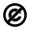# BIOINF 501 001 FA 2021

## BIOINF 501 001 FA 2021

Fall 2021 BIOINF 501

Mathematical Foundations for Bioinformatics

Overview

The course covers some of the fundamental mathematical techniques commonly used in bioinformatics and  biomedical research. These include: 1) principles of multi-variable calculus, and complex numbers/functions, 2) foundations of linear algebra, such as linear spaces, eigen-values and vectors, singular value decomposition, spectral graph theory and Markov chains, 3) differential equations and their usage in biomedical system, which includes topic such as existence and uniqueness of solutions, two dimensional linear systems, bifurcations in one and two dimensional systems and cellular dynamics, and 4) optimization methods, such as free and constrained optimization, Lagrange multipliers, data denoising using optimization and heuristic methods. Demonstrations using MATLAB, R, and Python will be included throughout the course.

All classes will be online via Zoom

Instructors
Kayvan Najarian, Shuyang Cheng, Reza Soroushmehr, and Ivo Dinov

TA: Da-Wei Lin

Start Time, End Time and Location

MW 1:30PM - 3:00PM,

Only the first session - Monday, August 31 - will be both in-person and online. All other sessions will be remote only.  Students are welcome to take the entire course online if they so choose.

Topics/Modules

Module 1: Review of Some Basic Methods in Mathematics

Probability functions

Review of complex variables and functions

Taught by: Kayvan Najarian

Duration: 3 lectures

Module 2: Linear Algebra

Introduction to linear systems

Matrix products

The inverse of a linear transformation

Linear spaces

Orthogonality

Determinants, eigenvalues and eigenvectors

Symmetric matrices and diagonalization

Solving systems of linear equations

Singular value decomposition

Principal component analysis

Spectral graph theory

Taught by: Shuyang Cheng

Duration: 9 lectures

Module 3: Differential Equations

Introduction to differential equations

First and second order linear equations

Existence and uniqueness of solutions

Difference equations

Systems of linear equations

Phase plane and bifurcation: diagrams and analysis

First order nonlinear systems

Differential equations for compartmental modeling of biomedical systems

Taught by: Reza Soroushmehr

Duration: 6 lectures

Module 4: Optimization

Data Science and Predictive Analytics EBook (University of Michigan Library)

Free (unconstrained) optimization vs. Constrained Optimization

Foundations of R

Equality and Inequality constraints

Lagrange Multipliers

Manual vs. Automated Lagrange Multiplier Optimization

Data Denoising: Application of computer optimization techniques in medicine and biology

Heuristic methods - Genetic algorithms, simulated annealing

Applications (supervised classification & unsupervised clustering)

Instructor: Ivo Dinov

Duration: 6 lectures

Software tools: Matlab, R, Python, and open-science tools.

## Course Summary:

Date Details DueThis course content is offered under a Public Domain license. Content in this course can be considered under this license unless otherwise noted.